# How can I get scaled cycloid curves?

#### strantor

Joined Oct 3, 2010
5,225
I'm trying to plot a cycloid curve, with a curve inside it and another one outside it, such that the inner curve and outer curve are equidistant along their entire length and the original curve lies between them.

Here is what I mean. I got the inner and outer curves in this picture by using a spline tool in CAD. I do not want to use a spline. I want to use an equation.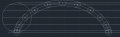I've tried every single modification to the original equation that I can think of, and I've resorted to just embarrassing randomness. I've been at this for many hours and I'm wielding mathematics like a monkey with a machine gun at this point. Figured it was time to ask for help.

#### strantor

Joined Oct 3, 2010
5,225
This is practical, not academic. There is no homework to show. But if it would help, I could show a handful of the hundreds of equations I've proven don't work.

#### Tesla23

Joined May 10, 2009
384
Assuming that the equation for a cycloid on Wikipedia is correct:

$x=r(t - sin t)$
$y = r(1-cos t)$

We want to find the equation of the curves that are a distance 'h' away at each point, moving at right angles to the tangent. So first find the direction of the tangent:

$dx/dt = r(1-cos t) = D_x$
$dy/dt = r sin t = D_y$

$t_x = \frac{D_x}{\sqrt{D_x^2 + D_y^2}}$
$t_y = \frac{D_y}{\sqrt{D_x^2 + D_y^2}}$

the unit tangent at a point is then $$(t_x, t_y)$$

At a point $$(x_0, y_0)$$ on the curve you want to move a distance $$h$$ at right angles to the tangent, so these two points are:

$(x_0 \pm t_y h, y_0 \mp t_x h)$

You can work out $$t_x$$ and $$t_y$$ in terms of t and substitute in, this will give you parameterised equations for the two curved (one using the top signs and the other using the lower signs)

#### cmartinez

Joined Jan 17, 2007
6,771
What you want is called an offset curve (at least in my part of the woods), and to derive its equation you need the original curve's equation first, and then at each of its points find its derivative (its slope), turn it into a linear equation, and then rotate it by plus and minus 90 degrees, and then add the offset distance you want.

I did some work on that quite a few years ago, and it worked perfectly that way.

#### MrAl

Joined Jun 17, 2014
7,139
I'm trying to plot a cycloid curve, with a curve inside it and another one outside it, such that the inner curve and outer curve are equidistant along their entire length and the original curve lies between them.

Here is what I mean. I got the inner and outer curves in this picture by using a spline tool in CAD. I do not want to use a spline. I want to use an equation.

View attachment 199641

I've tried every single modification to the original equation that I can think of, and I've resorted to just embarrassing randomness. I've been at this for many hours and I'm wielding mathematics like a monkey with a machine gun at this point. Figured it was time to ask for help.
Hi,

It is a little unclear what you want to do here to me.
If you want to show a cycloid of some type then you should at least show two graphic images so we can see what it is you want to generate. All i see now are three ellipses with small circles and one bigger circle with no explanation of how they got there.
So try showing at least two drawings, one drawing a partial drawing of what you want, then another drawing of a next phase of the cyclic process. The more stages of the overall graphic drawing you show the better.

#### atferrari

Joined Jan 6, 2004
3,780
I spent some time revising the concept of throchoids. Have you explored the curtate / prolate?

At first sight they seem insufficient to provide the last part reaching the x axis.

The very first graphic here is enough to see what I say.

#### strantor

Joined Oct 3, 2010
5,225
Assuming that the equation for a cycloid on Wikipedia is correct:

$x=r(t - sin t)$
$y = r(1-cos t)$

We want to find the equation of the curves that are a distance 'h' away at each point, moving at right angles to the tangent. So first find the direction of the tangent:

$dx/dt = r(1-cos t) = D_x$
$dy/dt = r sin t = D_y$

$t_x = \frac{D_x}{\sqrt{D_x^2 + D_y^2}}$
$t_y = \frac{D_y}{\sqrt{D_x^2 + D_y^2}}$

the unit tangent at a point is then $$(t_x, t_y)$$

At a point $$(x_0, y_0)$$ on the curve you want to move a distance $$h$$ at right angles to the tangent, so these two points are:

$(x_0 \pm t_y h, y_0 \mp t_x h)$

You can work out $$t_x$$ and $$t_y$$ in terms of t and substitute in, this will give you parameterised equations for the two curved (one using the top signs and the other using the lower signs)
Thank you. I think you're saying the same thing as @cmartinez but I lack the fundamentals to understand precisely what you're saying. It will help if the terms in your equations are defined. I will make an attempt to define them; please correct me.
t = angle of rotation of the rolling circle
r = radius of the rolling circle
d = ??
D = ??
h = radius of a smaller circle moving along the path of the cycloid

This example is how far I can work your equations with my current knowledge:
r = 4
t = 0.5*PI
h = 0.5

$x=4(.5π - sin .5π)=6.1735$
$y = 4(1-cos .5π)=0.0015$

So just the base cycloid, which I already can do.

#### strantor

Joined Oct 3, 2010
5,225
What you want is called an offset curve (at least in my part of the woods), and to derive its equation you need the original curve's equation first, and then at each of its points find its derivative (its slope), turn it into a linear equation, and then rotate it by plus and minus 90 degrees, and then add the offset distance you want.

I did some work on that quite a few years ago, and it worked perfectly that way.
Thanks. I've managed to make this work mostly, albeit in probably the most obviously (to any mathematician) laughably hacked way. I'm currently using matplotlib in Python to do it; focusing for clarity's sake on just the inner offset curve. Here is the result: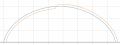Here is my code:

Python:
import matplotlib.pyplot as plot
import numpy as np

# Variable Parameters to be input
pitchDiameter = 6 # Diameter of the rolling circle
rollerDiameter = .75 # Diameter of a smaller circle centered on the focal point of the rolling circle
noOfRollers = 6 # Ignore for now

# constants (DON'T CHANGE)
pi = np.pi
# calculated variables (DON'T CHANGE)
pitchCircumference = pitchDiameter * pi
rollerCircumference = rollerDiameter * pi

# Get X/Y Values for basic cycloid curve
# create a numpy array containing radian values of a complete revolution of the circle in increments of .1
rotationAngle = np.arange(0, 2*pi, 0.1)
xValues = (pitchRadius * (rotationAngle - np.sin(rotationAngle))) # create a numpy array containing x values of the cycloid
yValues = pitchRadius * (1 - np.cos(rotationAngle))# create a numpy array containing y values of the cycloid
# Plot the basic cycloid curve
plot.plot(xValues, yValues)

# Create 2 lists to contain the x & y values of the inner offset curve.
innerXvalues = []
innerYvalues = []
# cycle through all values of rotation angles and find the equations of the line segments which make up the cycloid
for i in range (0,len(rotationAngle)-1):
# Find equation of the line between this point and the next point
X1,Y1 = xValues[i], yValues[i] # this point
X2,Y2 = xValues[i + 1], yValues[i + 1] # next point
M = (Y2-Y1)/(X2-X1) # Find the slope using the two points
# we now have all the values for the y=mx+b equation which describes this segment of the cycloid curve
# now we need to find a line perpendicular to this line segment and a point on that line a given distance away
innerB = np.arctan(1 / M)  # inverse slope (in radians) is the tangent of the cycloid
innerXvalues.append(innerX)
innerYvalues.append(innerY)
plot.plot(innerXvalues,innerYvalues)

plot.title('Cycloid Rack Profile')

plot.grid(True, which='both')

plot.axhline(y=0, color='k')

plot.show()
As you can see, as the radians approach PI, the offset jumps to the outside of the curve. I turned it into a piecewise formula to correct it.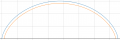Python:
    if rotationAngle[i] < 3.1:
else:
innerY = Y1 + np.sin(innerB)*rollerRadius

Thanks for the help!

P.S. The new code insertion tool is pretty sweet.

#### Attachments

•xox, atferrari and cmartinez

#### strantor

Joined Oct 3, 2010
5,225
If anyone is curious what this is all about, more details can be found here. I'm designing a rack & pinion system based on rollers instead of involute gear teeth, that hopefully will allow significant force of the pinion into the rack without binding, and also have zero backlash.

•cmartinez and atferrari

#### Tesla23

Joined May 10, 2009
384
Thanks. I've managed to make this work mostly, albeit in probably the most obviously (to any mathematician) laughably hacked way. I'm currently using matplotlib in Python to do it; focusing for clarity's sake on just the inner offset curve. Here is the result:
That looks fine - you've done numerically what I did analytically.

You can make it as accurate as you like by increasing the number of points. A simple way to estimate the error is to halve the interval and look at the points that are calculated in both sets, you will be able to see the convergence.

#### Tesla23

Joined May 10, 2009
384
here's the analytical solution:

For the cycloid
$x=r(t-sin(t))$
$y=r(1-cos(t))$
the equation of the curve offset a distance 'h' is surprisingly simple:
$x=r(t-sin(t))+h cos(t/2)$
$y=r(1-cos(t))-h sin(t/2)$

example for r=2, h=0.1, red curve is offset inside the cycloid:for h=-0.1: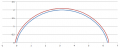•strantor and cmartinez

#### Tesla23

Joined May 10, 2009
384
This reduced to something so simple in the end there had to be a simple geometric explanation:

Apparently Descartes proved back in 1638 that the tangent to the cycloid is perpendicular to the line back to the point of contact of the rolling circle (so why was I doing calculus?). With a bit of geometry you can show that this line forms an angle of t/2 with the x axis - hence the formulas above. So essentially, to make the offset curve, you want to move along the line drawn from the point on the cycloid back to the point of contact - that will give you the equations in my last post.

•strantor

#### cmartinez

Joined Jan 17, 2007
6,771
If anyone is curious what this is all about, more details can be found here. I'm designing a rack & pinion system based on rollers instead of involute gear teeth, that hopefully will allow significant force of the pinion into the rack without binding, and also have zero backlash.
Did you do the proper geometric calculations to make sure that motion remains linear during the pinion's rotation?

#### strantor

Joined Oct 3, 2010
5,225
Did you do the proper geometric calculations to make sure that motion remains linear during the pinion's rotation?
No, sir. If it's not obvious, I'm not smart enough for that LOL. I planning to just make it and see if it works.

#### strantor

Joined Oct 3, 2010
5,225
Well I'm pretty happy with this. Later I'll add a function to export the x,y coords to a CSV which I can import into CAD/CAM to mill out the rack.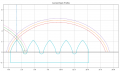Python:
import matplotlib.pyplot as plot
import numpy as np

# Variable Parameters to be input
pitchDiameter = 6 # Diameter of the rolling circle
rollerDiameter = .75 # Diameter of a smaller circle centered on the focal point of the rolling circle
noOfRollers = 6 # number of rollers (teeth) in pinion
noOfTeeth = 5 # desired number of teeth in rack assembly
webThickness = 2 # distance from center of roller at lowest point, and bottom edge of rack

# constants (DON'T CHANGE)
pi = np.pi
# calculated variables (DON'T CHANGE)
pitchCircumference = pitchDiameter * pi
rollerCircumference = rollerDiameter * pi

# create lists of X&Y values for the final rack tooth profile. We will add to these lists at various points along the process.
finalXvalues = []
finalYvalues = []

# this is a vertical line where the positive and the negative cycloids converge
xIntercept = (pitchCircumference/noOfRollers)/2
plot.plot([xIntercept,xIntercept],[0,10], '--')

# Get X/Y Values for basic cycloid curve
# create a numpy array containing radian values of a complete revolution of the circle in increments of .1
rotationAngle = np.arange(0, 2*pi, 0.1)
#print(rotationAngle)
xValuesA = (pitchRadius * (rotationAngle - np.sin(rotationAngle))) # create a numpy array containing x values of the cycloid
yValuesA = pitchRadius * (1 - np.cos(rotationAngle))# create a numpy array containing y values of the cycloid
# Plot the basic cycloid curve
plot.plot(xValuesA, yValuesA, '--') # draw a dashed line for visual reference only

# second cycloid is offset in the X axis by the period of the roller interval and in the negative direction
def offsetSecondCycloid(input):
return -input + (pitchCircumference / noOfRollers)
xValuesB = offsetSecondCycloid(xValuesA)
yValuesB = yValuesA
plot.plot(xValuesB, yValuesB, '--') # draw a dashed line for visual reference only

# Create 2 lists to contain the x & y values of the inner offset curve.
innerXvaluesA = []
innerYvaluesA = []
outerXvaluesA = []
outerYvaluesA = []
innerXvaluesB = []
innerYvaluesB = []
outerXvaluesB = []
outerYvaluesB = []
# cycle through all values of rotation angles and find the equations of the line segments which make up the cycloid
for i in range (0,len(rotationAngle)-1):
# Find equation of the line between this point and the next point
X1A, Y1A = xValuesA[i], yValuesA[i] # this point
X2A, Y2A = xValuesA[i + 1], yValuesA[i + 1] # next point
M = (Y2A - Y1A) / (X2A - X1A) # Find the slope using the two points
# we now have all the values for the y=mx+b equation which describes this segment of the cycloid curve
# now we need to find a line perpendicular to this line segment and a point on that line a given distance away
tangentSlope = np.arctan(1 / M)  # inverse slope (in radians) is the tangent of the cycloid
if rotationAngle[i] < 3.1:
innerX = X2A + np.cos(tangentSlope) * rollerRadius
innerY = Y2A - np.sin(tangentSlope) * rollerRadius
outerX = X2A - np.cos(tangentSlope) * rollerRadius
outerY = Y2A + np.sin(tangentSlope) * rollerRadius
else:
innerX = X1A - np.cos(tangentSlope) * rollerRadius
innerY = Y1A + np.sin(tangentSlope) * rollerRadius
outerX = X2A + np.cos(tangentSlope) * rollerRadius
outerY = Y2A - np.sin(tangentSlope) * rollerRadius
innerXvaluesA.append(innerX)
innerYvaluesA.append(innerY)
outerXvaluesA.append(outerX)
outerYvaluesA.append(outerY)
innerXvaluesB.append(offsetSecondCycloid(innerX))
innerYvaluesB.append(innerY)
outerXvaluesB.append(offsetSecondCycloid(outerX))
outerYvaluesB.append(outerY)

plot.plot(innerXvaluesA, innerYvaluesA, '--')
plot.plot(outerXvaluesA, outerYvaluesA,'--')
plot.plot(innerXvaluesB, innerYvaluesB,'--')
plot.plot(outerXvaluesB, outerYvaluesB,'--')
for i in range(0, len(innerYvaluesA)):
if innerXvaluesA[i] < xIntercept:
finalXvalues.append(innerXvaluesA[i])
finalYvalues.append(innerYvaluesA[i])
innerXvaluesB.reverse()
innerYvaluesB.reverse()
for i in range(len(innerYvaluesB)):
print(i)
if innerXvaluesB[i] > xIntercept:
finalXvalues.append(innerXvaluesB[i])
finalYvalues.append(innerYvaluesB[i])

for i in range (0,len(finalYvalues)):
print(finalXvalues[i],finalYvalues[i])
# Plot a circle representing the roller, at the end of the first downward slope
# formula of a circle: (x-h)^2 + (y-k)^2 = r^2, where
# h,k is the center of the circle and r is the radius
# since the circle is centered at unk,0, it is simply:
# (x-h)^2 + y^2 = r^2
# Rearrange for X
# (x-h)^2 = r^2 - y^2
# x - h = sqrt(r^2 - y^2)
# x = sqrt(r^2 - y^2) + h
rollerCircleXvalues = []
rollerCircleYvalues = []
h = (pitchCircumference / noOfRollers)
if n >= 0 and n < 0.5*pi:
elif n >= 0.5*pi and n < 1.5* pi:
rollerCircleXvalues.append(-np.sqrt(rollerRadius ** 2 - rollerCircleYvalues[i] ** 2) + h)
else:
rollerCircleXvalues.append(np.sqrt(rollerRadius ** 2 - rollerCircleYvalues[i] ** 2) + h)
plot.plot(rollerCircleXvalues, rollerCircleYvalues, '--')

finalXvalues.append(rollerCircleXvalues[i])
finalYvalues.append(rollerCircleYvalues[i])

###################################################################################
######## DUPLICATE FINAL X & Y VALUES BY # OF ROLLERS##############################
###################################################################################

rackXcoords = []
rackYcoords = []
rackYcoords.append(0)
for i in range (0,noOfTeeth):
if i < noOfTeeth-1:
for j in range(0,len(finalXvalues)):
rackXcoords.append(finalXvalues[j]+((pitchCircumference/noOfRollers)*i))
rackYcoords.append(finalYvalues[j])
else:
for j in range(0, len(finalXvalues)):
if finalYvalues[j] >= 0:
rackXcoords.append(finalXvalues[j] + ((pitchCircumference / noOfRollers) * i))
rackYcoords.append(finalYvalues[j])
finalVertice = rackXcoords[len(rackXcoords)-1]
rackXcoords.append(finalVertice)
rackYcoords.append(-webThickness)
rackYcoords.append(-webThickness)
rackYcoords.append(0)

plot.plot(finalXvalues,finalYvalues)
plot.plot(rackXcoords,rackYcoords)
plot.xlim(-1)

plot.title('Cycloid Rack Profile')

plot.grid(True, which='both')

plot.axhline(y=0, color='k')

plot.show()

#### strantor

Joined Oct 3, 2010
5,225
here's the analytical solution:

For the cycloid
$x=r(t-sin(t))$
$y=r(1-cos(t))$
the equation of the curve offset a distance 'h' is surprisingly simple:
$x=r(t-sin(t))+h cos(t/2)$
$y=r(1-cos(t))-h sin(t/2)$

example for r=2, h=0.1, red curve is offset inside the cycloid:
View attachment 199821

for h=-0.1:

View attachment 199822
That's so simple! UGH! Both frustrating and satisfying at the same time. When I go back to clean up my code (already previously vomited) I will change to your equations for neatness' sake. Thank you for figuring that out!

#### strantor

Joined Oct 3, 2010
5,225
I've implemented a new feature in my script that turns the vertices into a LWPolyLine, and saves it as a DXF file which I can directly import into AutoCAD/Inventor/Etc.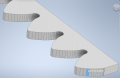From this perspective it is much more obvious the result of plotting this as line segments. I need to increase the resolution, and I will, but I want to understand better the workings of CAD/CAM/CNC. @cmartinez, @shortbus I think you're probably uniquely qualified to answer...

When shapes are described parametrically in CAD (ex: a circle with a radius of 3"), I do not see the polygon lines like I see in my rack part above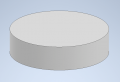It leads me to believe that a curve described by an equation is much more accurate than a curve described by coordinates. More specifically, it leads me to believe that a curve described by an equation is perfect, as it contains an infinite number of coordinates, and my script-generated rack part is something very far short of perfect, and always will be no matter the resolution, as a function of how it's derived.

However, given my only-slightly-better-than-layman's understanding of how CAM/CNC works, I believe that even in the case of the "perfect" circle, at some point between the computer screen and the endmill, this equation is turned into a set of coordinates. At some point, there is generated some "point A" and "point B." There has to be, otherwise how does the machine know how to go from point A to point B? So what is the resolution of that? And where does the translation occur? Or am I totally wrong? Does a CNC machine directly trace its paths according trig functions, to whatever degree of accuracy it's physically capable of?

I ask, mainly because I simply want to understand, but also because I perceive that there will be a point of diminishing returns as I increase the resolution; the point at which the resolution of my line is greater than the resolution of whatever orders the CNC is following, and to increase further would only slow down my computer.

•cmartinez

#### cmartinez

Joined Jan 17, 2007
6,771
I've implemented a new feature in my script that turns the vertices into a LWPolyLine, and saves it as a DXF file which I can directly import into AutoCAD/Inventor/Etc.

View attachment 199867

From this perspective it is much more obvious the result of plotting this as line segments. I need to increase the resolution, and I will, but I want to understand better the workings of CAD/CAM/CNC. @cmartinez, @shortbus I think you're probably uniquely qualified to answer...

When shapes are described parametrically in CAD (ex: a circle with a radius of 3"), I do not see the polygon lines like I see in my rack part above

View attachment 199868

It leads me to believe that a curve described by an equation is much more accurate than a curve described by coordinates. More specifically, it leads me to believe that a curve described by an equation is perfect, as it contains an infinite number of coordinates, and my script-generated rack part is something very far short of perfect, and always will be no matter the resolution, as a function of how it's derived.

However, given my only-slightly-better-than-layman's understanding of how CAM/CNC works, I believe that even in the case of the "perfect" circle, at some point between the computer screen and the endmill, this equation is turned into a set of coordinates. At some point, there is generated some "point A" and "point B." There has to be, otherwise how does the machine know how to go from point A to point B? So what is the resolution of that? And where does the translation occur? Or am I totally wrong? Does a CNC machine directly trace its paths according trig functions, to whatever degree of accuracy it's physically capable of?

I ask, mainly because I simply want to understand, but also because I perceive that there will be a point of diminishing returns as I increase the resolution; the point at which the resolution of my line is greater than the resolution of whatever orders the CNC is following, and to increase further would only slow down my computer.
As far as I know, standard G-code only does linear and arc interpolations when tracing cuts/motion. I am not aware of CNC code that performs any equation-based (such as splines) motion. And if it did, I would not be surprised if it broke it down into line segments (with resolution defined by the user) which would be later traced into a single interpolated smooth motion, depending on the particular controller. Maybe @MaxHeadRoom would be better suited to elaborate or add on what I'm saying.

Anyway, there is a function in AutoCAD called "flatten" which breaks down spline entities into polylines with straight and arc segments (the result being a very close approximation of the original), which in turn are perfectly translatable into G-code. Look it up.

Also, it would be quite helpful for me if you were to post the file drawing you're working on so I could take a look.

•strantor